# Difference / Ratio Problems Are as Easy as ABC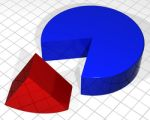Many students lose marks at the last part of Section C, where each question is worth 3 to 5 marks. It is this section that differentiates excellent (A*) students from the good (A) ones. About 3 of 10 questions are on ratio and difference problems, so it’s crucial to know how to solve these problems.

Instead of using the more complicated algebra and simultaneous equation method, I will show you a very simple heuristic solution. This means I break the problem into smaller steps and draw out models so that you can visualize the solution.

### Identifying the problem

The first step in approaching the problem is to identify if it is a difference and ratio problem.

Almost always, ratio and difference problems have two or more groups of items, in a specific ratio or difference. An action is performed (either by subtraction /addition of a quantity from one or both groups) and the ratio or difference changes. Then you will have to solve for either the quantity of the items in one or both groups, before or after the action.

Examples:

There were 112 fewer red apples than green apples in a basket. Ken sold 14  of the red apples and 38  of the green apples. The number of green apples left was twice as many as the number of red apples left. How many apples were there in the basket at first?

There are two groups: red apples and green apples. The difference is 112 apples. The action is selling 14 red and 38 apples. The end ratio is 1:2. Solve for total number of apples at first.

Roger had some fairy lights. He used 1/3  of the fairy lights to decorate 15 small Christmas trees and 2/5  of the fairy lights to decorate 6 big Christmas trees. He kept the rest. Each big Christmas tree had 14 more fairy lights than each small Christmas tree. How many fairy lights did Roger have at first?

Two groups: small Christmas trees and big Christmas trees. Difference between big and small is 14. Action is using 1/3 of his lights for small and 2/5 of his lights for the big trees. Solve for total number of lights.

At a swimming meet, School A had 18 more representatives than School B and 6 fewer   representatives than School C. The ratio of the number of boys to girls from the three schools was 1 : 3. The ratios of the number of boys to the number of girls in School A, B and C were 1 : 3, 1 : 5 and 2 : 5 respectively. How many representatives from the three schools were there in all?

Three groups: A, B and C. Differences are 18 and 6. Ratio is 1:3, 1:5 and 2:5. Action is to add all the boys and girls together to give a ratio of 1:3. Solve for total number of representatives.

Once you have seen enough of these ratio and difference problems, the identification will take less than a few seconds.

### The steps

Here is the mechanical model drawing steps to solving these problems.:

1. When you see group A has a number of items more (or less) than group B, you can draw a model representing both A and B with a number appended to group A. For example: “School A had 18 more representatives than School B”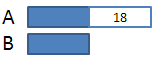2. When you see fractions or ratios on the same amount, work with a common denominator. Also, because it’s easier to work with whole numbers than fractions, you can multiply the fractions by the common denominator wherever possible. For example: “Roger used 1/3  of the fairy lights to decorate 15 small Christmas trees and 2/5  of the fairy lights to decorate 6 big Christmas trees.” In this case 1/3 = 5/15 and 2/5 = 6/15. However instead of working with 5/15 and 6/15 you can instead multiply the fractions by 15 and work with 5U and 6U.

3. Divide the boxes into corresponding units when you see ratios or fractions. Sometimes it may be easier to draw 4 or 5 more boxes than to divide the boxes into 4 or 5. Using the same example above, we can draw the 15 small Christmas trees into 5 boxes and the 6 big Christmas trees into 6 boxes.

4. Fill up as much information as you can from the question into the model diagram. When possible, try to reduce the number of unknowns as you work through the diagram.

5. Try to equate the before and after together, by incorporating the “action” in the diagram.

6. Solve for 1U and answer the question.

### Worked examples (from simple to harder):

Here are some worked examples to show these steps in action:

Sue had \$80 more than her brother. After Sue spent \$25 of her money and her brother spent 75% of his money, they still had \$420 left altogether.  How much did Sue have left in the end?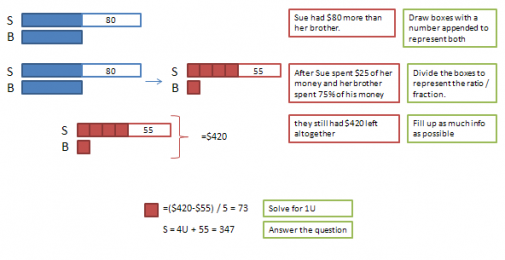There were 112 fewer red apples than green apples in a basket. Ken sold 14  of the red apples and 38  of the green apples. The number of green apples left was twice as many as the number of red apples left. How many apples were there in the basket at first?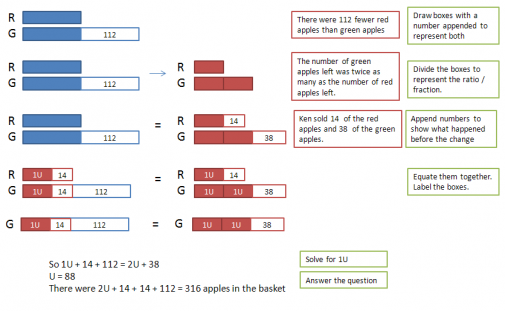Roger had some fairy lights. He used 1/3  of the fairy lights to decorate 15 small Christmas trees and 2/5  of the fairy lights to decorate 6 big Christmas trees. He kept the rest. Each big Christmas tree had 14 more fairy lights than each small Christmas tree. How many fairy lights did Roger have at first?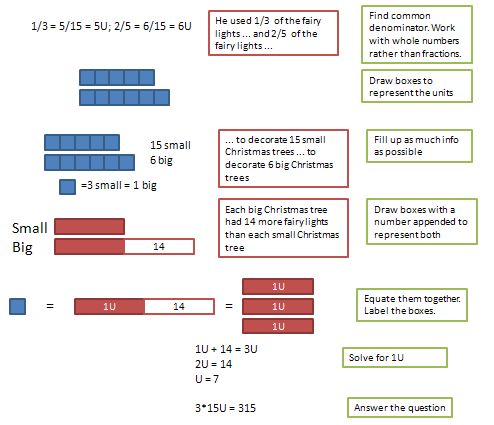Cindy, Dick and Eddy had \$410 altogether. Cindy gave 1/3 of her money to Eddy. Dick then gave 2/5 of his money to Cindy. As a result, Dick had \$20 less than Cindy and \$30 less than Eddy. How much money had Cindy had at first?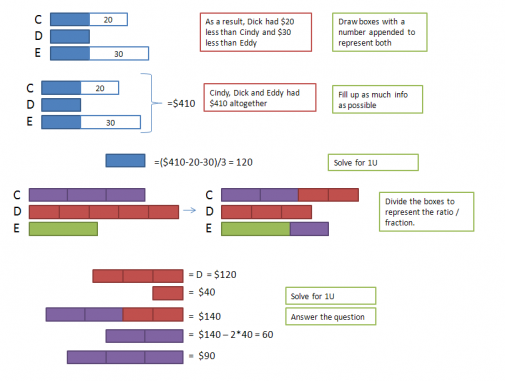There were 156 more pupils in the playground than the library. 1/5 of the pupils in the library were girls and 5/6 of the pupils in the playground were boys. If there were 17more girls in the playground than the library. Find the total number of pupils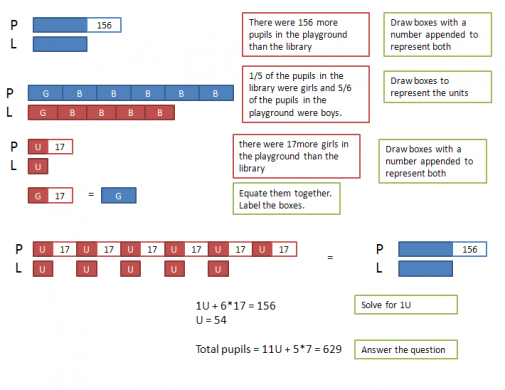At a swimming meet, School A had 18 more representatives than School B and 6 fewer   representatives than School C. The ratio of the number of boys to girls from the three schools was 1 : 3. The ratios of the number of boys to the number of girls in School A, B and C were 1 : 3, 1 : 5 and 2 : 5 respectively. How many representatives from the three schools were there in all?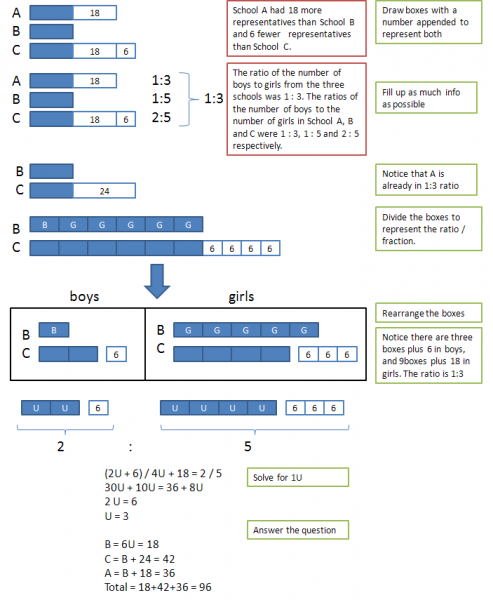As you can see, these ratio and difference problems are not that difficult. With a sufficient amount of practice and the right method, it’s as easy as ABC.

Wallace is a trader who has recently started a Facebook site with his buddy to help PSLE queries. Like them here http://facebook.com/studyroomjr

17 Likes

# I see. I understand now.

I see. I understand now. thanks PSLEguru.

# re: mathsnoobs

Hi,

From the 5 blue boxes = 15 small trees we find that 1 blue box = 3 small trees

From the 6 blue boxes = 6 big trees we find that 1 blue box = 1 big tree.

so 1 big = 3 small.

# Question

For the 1st question, how do you get 1 small = 3 big ?

# re: mathsnoobs

Hi mathsnoobs,

For the first query, indeed you are correct. The total number of fairy lights should be 3x that number. 1U represents 1 small Christmas tree and 3U represents 1 blue box. so 15x3U is the total number of lights. I have corrected the post for future reference.

For the second query, take a look at school B first of all. After breaking it into boys and girls (1 boy vs 5 girls) we are left with school C to break up. We break the 24 students into the 1:3 ratio and we are left with 6 blocks. But now we notice that girls already has 5 blocks and boys has 1 block. In order to maintain that 1:3 ratio, we place 2 blocks with boys and 4 blocks with girls. So boys have three blocks + 6 and girls have 9 blocks + 18. This maintains the 1:3 ratio.

Now we know school C is in the 2:5 ratio. so we can use 2:5 -> 2u+6 : 4u + 18.

A is already in the 1:3 ratio so it doesn’t need to be considered. It doesn’t matter if the 6 units cannot be split into 1:3 because its already in the correct ratio. Of course you can consider it but it will make the solution more complicated.

Hope it helps!

# I’m afraid I don’t understand

I’m afraid I don’t understand the solution to this question:

Roger had some fairy lights. He used 1/3​  of the fairy lights to decorate 15 small Christmas trees and 2/5​  of the fairy lights to decorate 6 big Christmas trees. He kept the rest. Each big Christmas tree had 14 more fairy lights than each small Christmas tree. How many fairy lights did Roger have at first?

1/3 of the fairy lights are used on 15 small Christmas trees.

Since the answer is 105, I’ll work from there.

3units = 105, 1unit = 35

So, each Small Christmas tree has 35/15  = 7/3 fairy lights

2/5 of the fairy lights are used on 6 big Christmas trees

5parts = 105, 2parts = 42

Each Big Christmas tree has 42/6 = 7 fairy lights

I don’t quite get this -> Each big Christmas tree had 14 more fairy lights than each small Christmas tree.

The difference between the Big Christmas tree and Small Christmas tree is 7 – 7/3 = 14/3 fairy lights. I don’t get 14 as stated in the question.

————————————————————–

the other problem I had is this question:

At a swimming meet, School A had 18 more representatives than School B and 6 fewer   representatives than School C. The ratio of the number of boys to girls from the three schools was 1 : 3. The ratios of the number of boys to the number of girls in School A, B and C were 1 : 3, 1 : 5 and 2 : 5 respectively. How many representatives from the three schools were there in all?

I don’t understand how this is derived:

School C-> Boys: Girls -> 2:5 -> 2u + 6 : 4u + 18

overall for School C there are 6 units of Boys and Girls. How do you break 6 units to 2:5 parts?

In your example, you used B and C to get 1:3. But 1:3 should incorporate A, B and C?

You’ve also mentioned that A is already in 1:3 ratio, but A has 6 units, I  don’t quite understand how 6 units is split into 1:3 parts